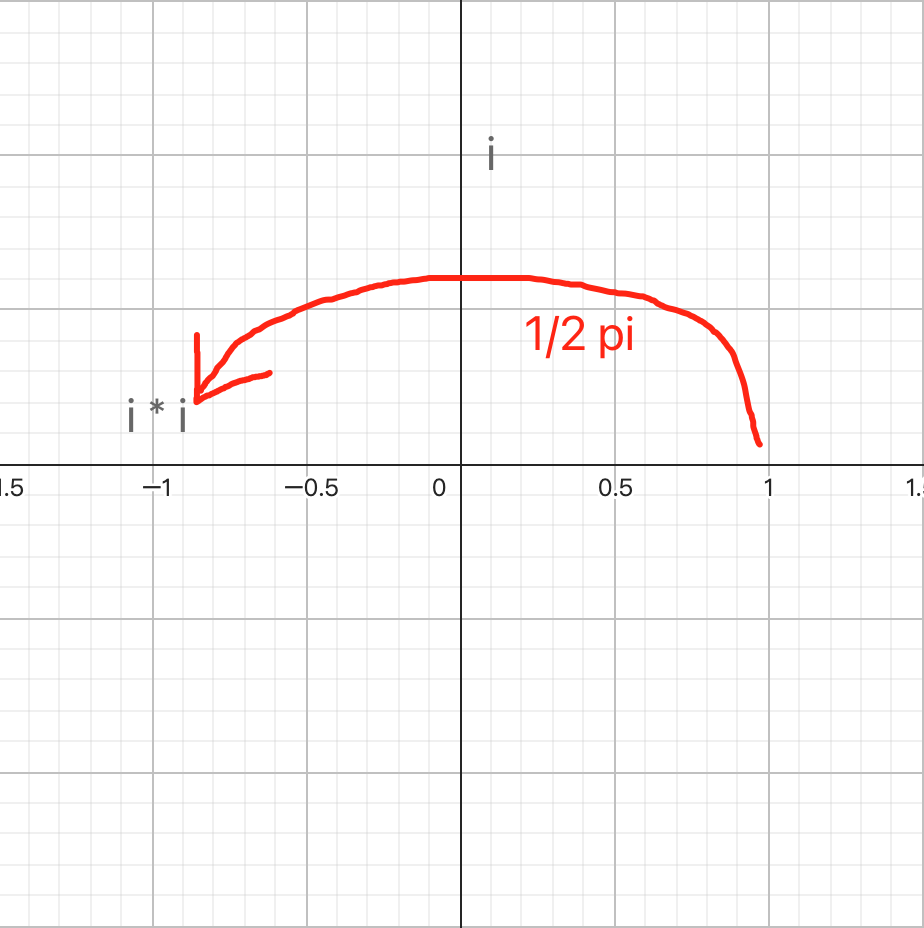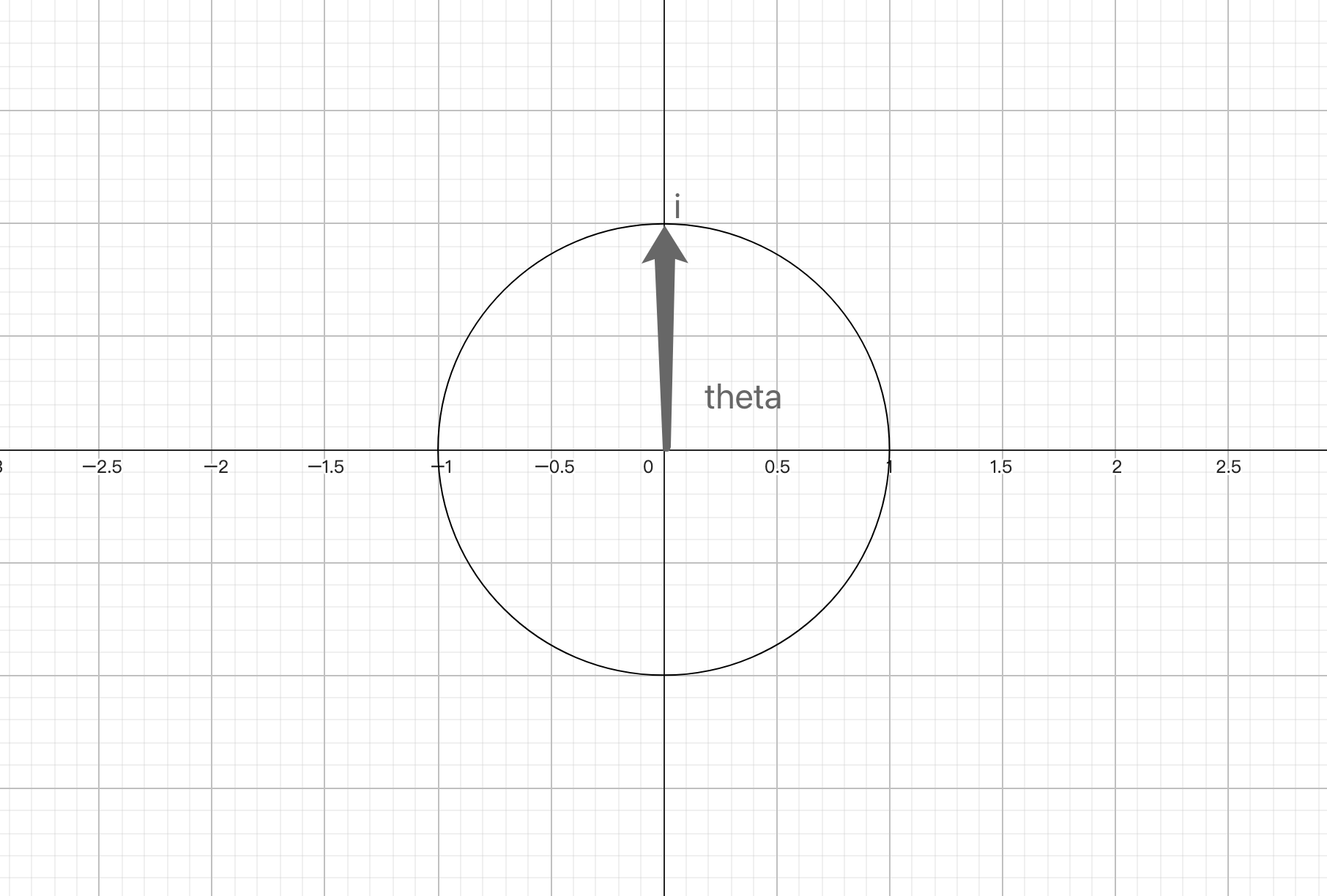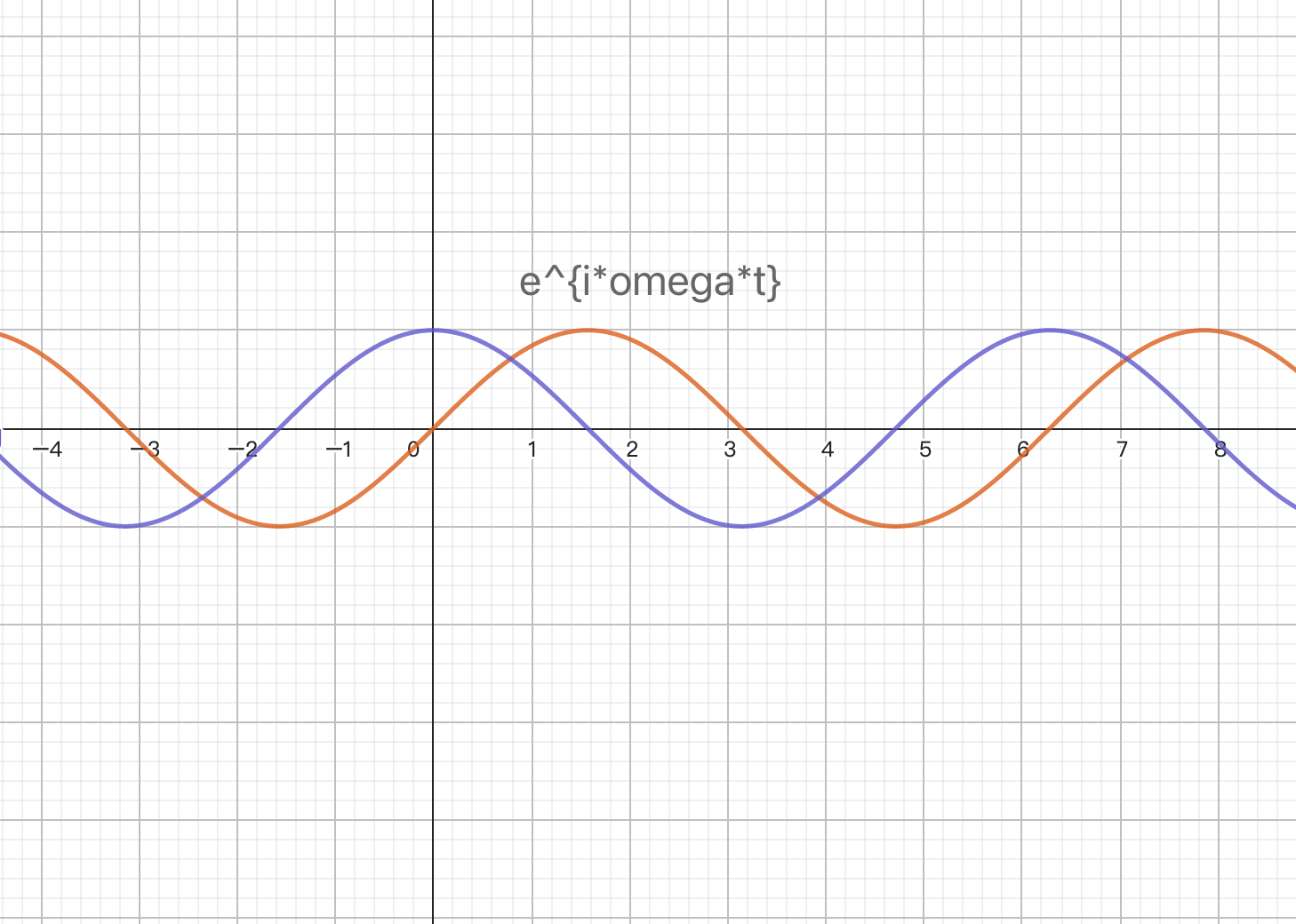• # 复平面计算本质和傅里叶变换

Author：张一极

date：2022年11月12日11:26:15

### 复平面

#### 先验知识：

0.欧拉公式：

$\begin{array}{}\text{(1)}& {e}^{i\theta }=\mathrm{cos}\theta +i\mathrm{sin}\theta \end{array}$

theta作为角度，可以换成跟时间有关的其他变量，比如${e}^{i\omega t}$$e^{i \omega t}$，可以表示一个圆心角随时间变化情况。

1.复数乘法：

一般式：$\left(a+bi\right)\left(c+di\right)=ac+adi+bci+bd{i}^{2}$$(a+b i)(c+d i)=a c+a d i+b c i+b d i^2$

2.根据欧拉公式复数乘法的极坐标系写法：

$\begin{array}{}\text{(2)}& \begin{array}{r}a+bi={r}_{1}\left(\mathrm{cos}{\theta }_{1}+i\mathrm{sin}{\theta }_{1}\right)\\ c+di={r}_{2}\left(\mathrm{cos}{\theta }_{2}+i\mathrm{sin}{\theta }_{2}\right)\end{array}\end{array}$

二者相乘得到：

$\begin{array}{}\text{(3)}& {r}_{1}{r}_{2}\left(\mathrm{cos}{\theta }_{1}+i\mathrm{sin}{\theta }_{1}\right)\left(\mathrm{cos}{\theta }_{2}+i\mathrm{sin}{\theta }_{2}\right)={r}_{1}{r}_{2}\left[\mathrm{cos}\left({\theta }_{1}+{\theta }_{2}\right)+i\mathrm{sin}\left({\theta }_{1}+{\theta }_{2}\right)\right]\end{array}$

即，复数极坐标中相乘可以表示为：幅角相加，模长相乘，所以这时候我们在推广到，单位复数的平方，即$i\cdot i=-1$$i\cdot i = -1$,其实就是因为，幅度相加，每一个i都在虚轴上一个单位长，一个i旋转90，两个i就旋转到180度，即-1。theta为幅角，i幅角为90:欧拉公式

$\begin{array}{}\text{(4)}& {e}^{i\pi }=\underset{n\to \mathrm{\infty }}{lim}{\left(1+\frac{i\pi }{n}\right)}^{n}=-1\end{array}$

以及：

$\begin{array}{}\text{(5)}& {e}^{i\theta }=\underset{n\to \mathrm{\infty }}{lim}{\left(1+\frac{i\theta }{n}\right)}^{n}\end{array}$

用复数${e}^{i\omega t}$$e^{i \omega t}$，表达旋转，或表达周期函数，可以表达任意频率，以及方向的周期函数。### 这时候的傅立叶变换

定义（来自维基百科）：Fourier transform integral

$\begin{array}{}\text{(6)}& \stackrel{^}{f}\left(\xi \right)={\int }_{-\mathrm{\infty }}^{\mathrm{\infty }}f\left(x\right){e}^{-i2\pi \xi x}dx,\phantom{\rule{1em}{0ex}}\mathrm{\forall }\xi \in \mathbb{R}\end{array}$

其中，$f\left(x\right)$$f(x)$为模长，${e}^{2\pi it}$$e^{2πit}$ 表示了一个点，以每秒一圈的频率，即频率为1绕着圆心旋转，那么${e}^{2\pi it\cdot \xi x}$$e^{2πit\cdot ξx}$就代表了一个点，顺时针旋转，频率为ξ，$x$$x$可以认为是时间，或者任意其他的变换量，它影响着总体旋转的点位，${e}^{-2\pi it\cdot \xi x}$$e^{-2πit\cdot ξx}$则表示一个频率为ξ，逆时针旋转的点，结合欧拉公式中，${e}^{i\omega t}$$e^{i \omega t}$可以认为，其中的$\omega =2\pi \xi$$\omega = 2\pi \xi$

傅里叶变换的本质是在说明，每一个复杂函数$f\left(\xi \right)$$f(ξ)$，都可以被n个$f\left(x\right){e}^{-i2\pi \xi x},\phantom{\rule{1em}{0ex}}\mathrm{\forall }\xi \in \mathbb{R}$$f(x) e^{-i 2 \pi \xi x} , \quad \forall \xi \in \mathbb{R}$组成，其表现形式即，把每一个分子做积分，从最小（负无穷）积到最大（正无穷）。